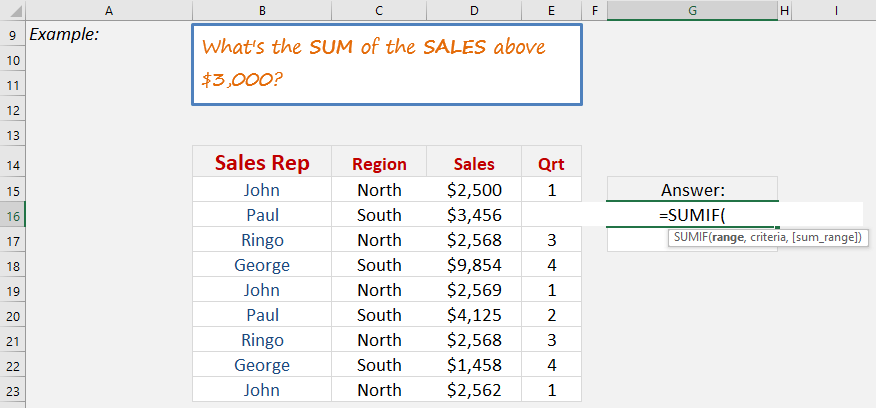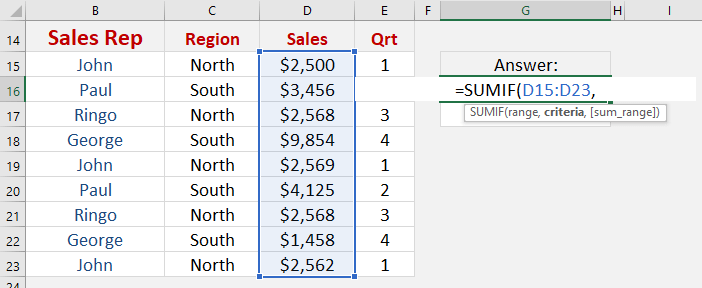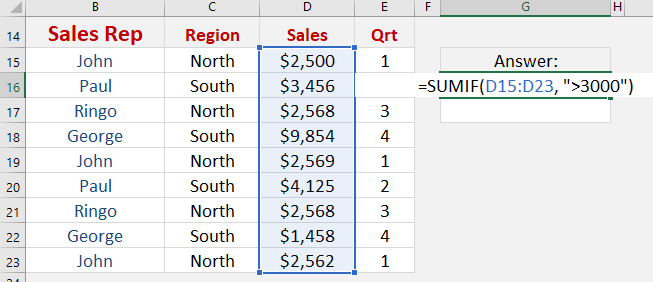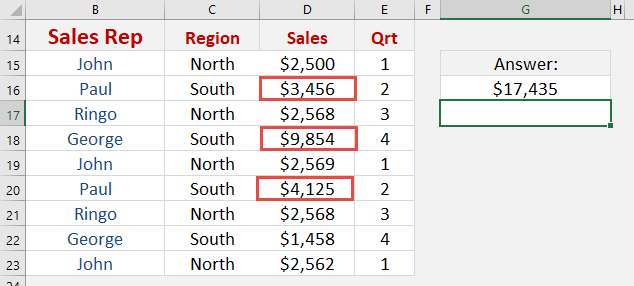What does it do?

Sums the values in a range that meet a criteria that you specify

Formula breakdown:

=SUMIF(Range or Cells, Criteria, [Sum_Range])

What it means:

=SUMIF(Evaluate this Range/Cells, With this Criteria, [Optional Sum Range])

The SUMIF function is used widely amongst spreadsheet users as it is a simple Excel function.  It allows you to Sum the values in a range that meet a criteria that you specify.

So if you want to Sum a range of sales values that are above \$3,000 then this is the best Excel function to use, as I explain below.We want to get the sum of the sales amounts that are above \$3000.

STEP 1: We need to enter the SUMIF function in a blank cell:

## =SUMIF(STEP 2: The SUMIF arguments:

## Range

What is your range that contains the source data?

Highlight the column that contains the sales data

## =SUMIF(D15:D23,## Criteria

Which records do you want to sum together?

Since we want to sum the amounts greater than 3000, then let’s type in >3000

## =SUMIF(D15:D23, “>3000”)Just like that, Excel has selectively found the values and summed them together!How to Use the Sumif Formula in Excel